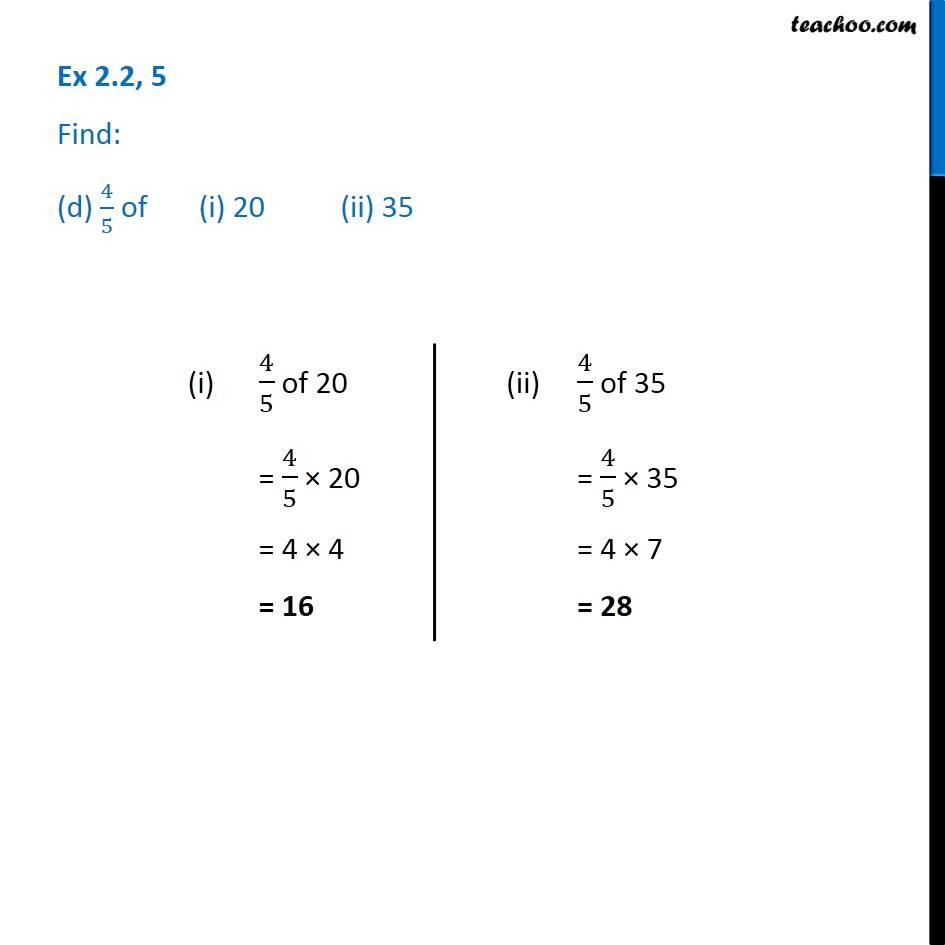1. Chapter 2 Class 7 Fractions and Decimals
2. Serial order wise
3. Ex 2.2

Transcript

Ex 2.2, 5 Find: (d) 4/5 of (i) 20 (ii) 35 (i) 4/5 of 20 = 4/5 × 20 = 4 × 4 = 16 (ii) 4/5 of 35 = 4/5 × 35 = 4 × 7 = 28